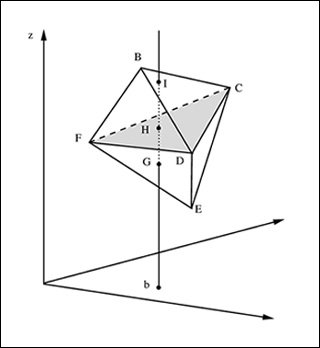MITIT & Software

# [100% Off] INTRODUCTION TO MATHEMATICAL PROGRAMMING Free Course Coupon

Updated: by ghatfanmerie

Click to get the course

8.0 hr
English
Free
Get the coupon in the end of description.

### Description

This course is an introduction to linear optimization and its extensions emphasizing the underlying mathematical structures, geometrical ideas, algorithms and solutions of practical problems. The topics covered include: formulations, the geometry of linear optimization, duality theory, the simplex method, sensitivity analysis, robust optimization, large scale optimization network flows, solving problems with an exponential number of constraints and the ellipsoid method, interior point methods, semidefinite optimization, solving real world problems problems with computer software, discrete optimization formulations and algorithms.
If the coupon is not opening, disable Adblock, or try another browser.